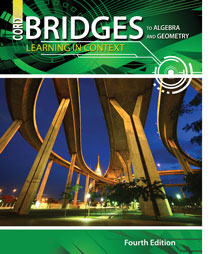# Bridges to Algebra and Geometry - 4th Edition

## Chapter 13: Surface Area and Volume

Some links are repeated for use with more than one lesson.

### 13.1  Drawing Three-Dimensional Figures

http://www.technologystudent.com/designpro/orthogrp1.html
http://www.technologystudent.com/prddes1/drawtec2.html
http://www.technologystudent.com/prddes1/persy2.html
NEW These three pages introduce orthographic, isometric, and perspective drawings with animated graphics. Much more is available if desired at the site index.

http://www.worksheetworks.com/miscellanea/graph-paper/isometric-dots.html
NEW This page will generate isometric dot paper you can print to aid in making isometric drawings of rectangular figures, as described in the text.

http://www.math.com/school/subject3/lessons/S3U4L1GL.html
This site defines figures in space and shows examples of each.

### 13.2  Surface Area of Prisms and Cylinders

http://www.math.com/school/subject3/lessons/S3U4L2GL.html
This site defines the different types of prisms and shows examples of each.

http://www.math.com/tables/geometry/surfareas.htm
This site defines the surface area of various shapes and gives their formula.

http://www.aaamath.com/g79-surface-area-cylinder.html
This site explains how to find the surface area of a cylinder and allows students to practice this concept.

http://www.mathsisfun.com/geometry/cuboids-rectangular-prisms.html
NEW This site shows how to calculate the surface area and volume of prisms, with practice problems.

http://www.regentsprep.org/Regents/math/ALGEBRA/AS2/indexAS2.htm
NEW This site gives formulas abd strategies for calculating the surface area and volumes of various objects, with examples and practice problems.

### 13.3  Surface Area of Prisms and Cylinders

http://www.math.com/tables/geometry/surfareas.htm
This site defines the surface area of various shapes and gives their formula.

http://www.mathsisfun.com/geometry/cylinder.html
NEW This site presents the geometry facts for cylinders, including practice problems.

http://www.mathsisfun.com/geometry/cylinder.html
This site gives definitions and practice problems that define cylinders.

### 13.4  Volume of Prisms and Cylinders

http://www.mathsisfun.com/geometry/prisms.html
NEW This site helps you identify a prism and determine its volume, including practice problems.

http://www.mathsisfun.com/geometry/polyhedron-models.html
NEW This site helps you visualize and identify the technical name of various prisms and polyhedrons.

http://www.math.com/school/subject3/lessons/S3U4L4GL.html
This site defines cylinders, cones, and spheres and shows examples of each.

### 13.5  Volume of Pyramids and Cones

http://www.math.com/school/subject3/lessons/S3U4L3GL.html
This site defines pyramids and shows examples of each.

http://www.math.com/tables/geometry/surfareas.htm
This site defines the surface area of various shapes and gives their formula.

http://www.mathsisfun.com/geometry/pyramids.html
NEW This site helps you identify a pyramid and calculate its volume and surface area, with practice problems.

http://www.mathsisfun.com/geometry/cone.html
NEW This site helps you identify a cone and calculate its volume and surface area, with practice problems

### 13.6  Similar Solids

http://teachers.henrico.k12.va.us/math/IGO/09AreaVolume/9-5SimilarObjects/LECTURE9-5.ppt
NEW This link is a small set of PowerPoint slides that present a lesson on the surface area and volume of similar solids.